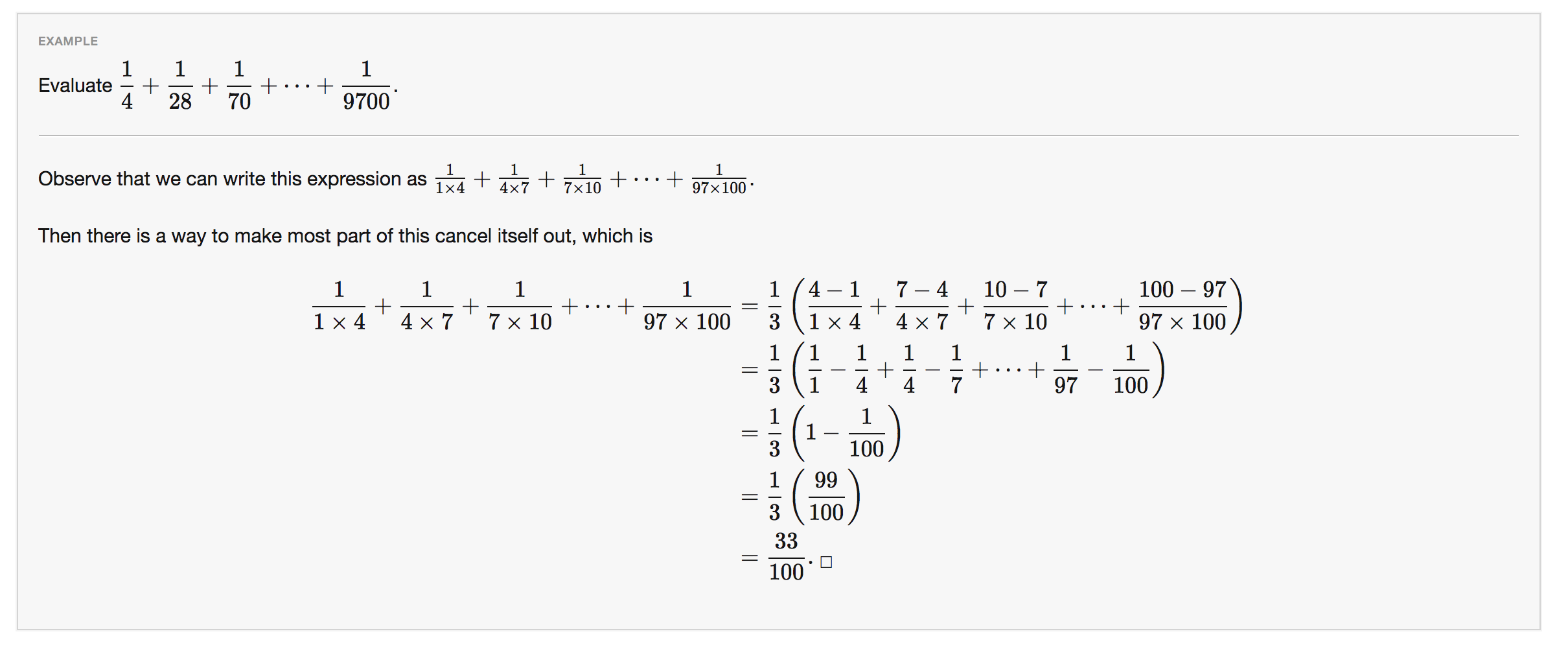# Help in understanding this...

On the wiki page for telescoping series, this example is given....Can someone help me understand the second step, where the fraction is reduced to all 1s in the numerator? I've researched the internet to understand how this was so simply done. How does (4-1)/(1*4) reduce so nicely? (I know it does, obviously), but there is a template here where the numerators go to 1 while the denominators go individually to, say, 1 and 4 (in the first fraction).

Is there a reason 1/3 was pulled out of the series? How was that number derived, other than trial and error.

It a general rule that (x-y)/(yx) can be reduced to 1/y minus 1/x. Is creating a situation where you have (x-y)/(yx) the basis for finding the 1/3 that is outside the parentheses and for how you go about solving this type of problem more generally?1 year, 11 months ago

This discussion board is a place to discuss our Daily Challenges and the math and science related to those challenges. Explanations are more than just a solution — they should explain the steps and thinking strategies that you used to obtain the solution. Comments should further the discussion of math and science.

When posting on Brilliant:

• Use the emojis to react to an explanation, whether you're congratulating a job well done , or just really confused .
• Ask specific questions about the challenge or the steps in somebody's explanation. Well-posed questions can add a lot to the discussion, but posting "I don't understand!" doesn't help anyone.
• Try to contribute something new to the discussion, whether it is an extension, generalization or other idea related to the challenge.

MarkdownAppears as
*italics* or _italics_ italics
**bold** or __bold__ bold
- bulleted- list
• bulleted
• list
1. numbered2. list
1. numbered
2. list
Note: you must add a full line of space before and after lists for them to show up correctly
paragraph 1paragraph 2

paragraph 1

paragraph 2

[example link](https://brilliant.org)example link
> This is a quote
This is a quote
    # I indented these lines
# 4 spaces, and now they show
# up as a code block.

print "hello world"
# I indented these lines
# 4 spaces, and now they show
# up as a code block.

print "hello world"
MathAppears as
Remember to wrap math in $$ ... $$ or $ ... $ to ensure proper formatting.
2 \times 3 $2 \times 3$
2^{34} $2^{34}$
a_{i-1} $a_{i-1}$
\frac{2}{3} $\frac{2}{3}$
\sqrt{2} $\sqrt{2}$
\sum_{i=1}^3 $\sum_{i=1}^3$
\sin \theta $\sin \theta$
\boxed{123} $\boxed{123}$

Sort by:

This follows by simply observing that

$\frac{4-1}{1\cdot4}=\frac{4}{1\cdot4}-\frac{1}{1\cdot4}=\frac{1}{1}-\frac{1}{4}$

The reason for taking out $\displaystyle \frac{1}{3}$ is for the numerators to get cancelled out...….here's an example...…

$\frac{1}{1\cdot7}+\frac{1}{7\cdot13}+\frac{1}{13\cdot19}+...$

$=\frac{1}{6}\left(\frac{6}{1\cdot7}+\frac{6}{7\cdot13}+\frac{6}{13\cdot19}+...\right)$

$=\frac{1}{6}\left(\frac{7-1}{1\cdot7}+\frac{13-7}{7\cdot13}+\frac{19-13}{13\cdot19}+...\right)$

$=\frac{1}{6}\left(\frac{7}{1\cdot7}-\frac{1}{1\cdot7}+\frac{13}{7\cdot13}-\frac{7}{7\cdot13}+\frac{19}{13\cdot19}-\frac{13}{13\cdot19}+...\right)$

$=\frac{1}{6}\left(\frac{1}{1}-\frac{1}{7}+\frac{1}{7}-\frac{1}{13}+\frac{1}{13}-\frac{1}{19}+...\right)$

$=\frac{1}{6}$

- 1 year, 11 months ago

Check out Partial Fractions to learn how to decompose such fractions.

Staff - 1 year, 11 months ago